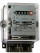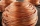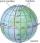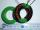# Ohm's law + fundamentals of Physics - math problems

#### Number of problems found: 16

• Cooker spiralA current of 4.4 A flow through the spiral of the electric cooker at a voltage of 220 V. What is the resistance of the spiral?
• ManganinHow long must a manganin wire with a diameter of 0.8 mm have to have a value of 10Ω?
• Direction indicatorAs that which the electrical voltage is connected to the lamp direction indicator 7400 mOhm resistance when current of 1.6 A passes through it?
• Circuit voltageCalculate the closed-circuit voltage. Calculate the power on the resistors. R1 = 30 ohms, R2 = 10 ohms. I = 0.1A. U =?. The resistors are connected in series, one after the other.
• Copper windingCalculate the current flowing through the copper winding at an operating temperature of 70°C. Celsius, if the winding diameter is 1.128 mm and the winding length is 40 m. The winding is connected to 8V.
• Current in the conductorCalculate the current in the conductor (in mA) if it is connected to a 4.5 V voltage source and its resistance is 20 (ohm).
• Two resistorsTwo resistors 20 Ω and 60 Ω are connected in series and an external voltage of 400 V is connected to them. What are the electrical voltages on the respective resistors? Please comment!
• Lowest voltageThree resistors with resistors R1 = 10 kΩ, R2 = 20 kΩ, R3 = 30 kΩ are connected in series and an external voltage U = 30 V is connected to them. On which resistor is the lowest voltage?
• TransformerSolve the textbook problems - transformer: a) N1 = 40, N2 = 80, U2 = 80 V, U1 =? b) N1 = 400, U1 = 200 V, U2 = 50 V, N2 =?
• R1+R2 in parallelThe resistance ratio of the first and second resistors is 3: 1 and the current flowing through the first resistor is 300 mA. What current flows through the second resistor (resistors connected in parallel)?
• Resistance - ohmsIf the resistance of the first resistor is 300 ohms and the resistance of the second resistor is 100 ohms. What is the resulting resistance?
• Serial connectionIf the voltage on the first resistor is 6 V and the resistance ratio of the first and second resistors is 1: 3, what will be the voltage across the second resistor if they are connected in series?
• Filament of bulbThe filament of bulb has a 1 ohm resistivity and is connected to a voltage 220 V. How much electric charge will pass through the fiber when the electric current passes for 10 seconds?
• Coil as a girlThe electrical resistance of the copper wire coil is 2.0 ohms. What current runs through the coil when the voltage between the terminals is 3.0 V?
• Resistance of the resistorThe resistor terminals have a voltage of 20 V and a current of 5 mA is passed through. What is the resistance of the resistor?
• ResistanceDetermine the resistance of the bulb with the current 200 mA and is in a regular lamp (230V).

Do you have an exciting math question or word problem that you can't solve? Ask a question or post a math problem, and we can try to solve it.

We will send a solution to your e-mail address. Solved examples are also published here. Please enter the e-mail correctly and check whether you don't have a full mailbox.

Ohm's law - math problems. Fundamentals of Physics - math problems.